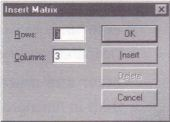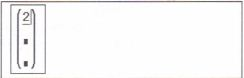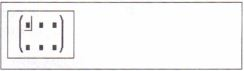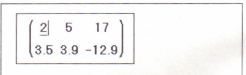# Vectors and Matrices MathCad Help

This chapter describes Mathcad arrays. While ordinary variables (scalars) hold a single value, arrays hold many values. As is customary in linear algebra, arrays having only one column will often be referred to as vectors. All others are matrices. The following sections make up this

Creating a vector or matrix
How to create or edit vectors and matrices

Computing with arrays
Defining variables as arrays and using them in expressions.

Subscripts and superscripts
Referring to individual array elements and columns.

Displaying vectors and matrices .

Limits on array sizes
Limits on the sizes of arrays to be stored, displayed, or entered.

Vector and matrix operators
Operators designed for use with vectors and matrices.

Vector and matrix functions
Built-in functions designed for use with vectors and matrices.

Doing calculations in parallel
Using Mathcad’s “vectorize” operator to speed calculations.

Simultaneous definitions
Using vectors to define several variables simultaneously.

Arrays and user-defined functions
Using arrays as arguments to user defined functions.

Nested arrays
Arrays in which the elements are themselves arrays.

Creating a vector or matrix

A single number in Mathcad is called a scalar. A column of numbers is a vector, and a rectangular array of numbers is called a matrix. The general term for a vector or matrix is an array

There are three ways to create an array

• By filling in an array of empty placeholders as discussed in this section. This technique is useful for arrays that are not too large.

• By using range variables to fill in the elements as discussed in Chapter 11, “Range Variables.” This technique is useful when you have some explicit formula for the elements in terms of their indices.

• By reading data in from external files or applications as discussed in Chapter 19, “Data Management,” and Chapter 28, “Importing and Exporting Graphics.”

You may wish to distinguish between the names of matrices, vectors, and scalars by font. For example, in many math and engineering books, names of vectors are set in bold while those of scalars are set in italic. See the section “Math styles” in Chapter 6 for a description of how to do this

Creating a vector

A vector is an array or matrix containing one column. To create a vector in Mathcad, follow these steps:

• Click in either a blank space or on a placeholder.• Choose Matrix from the Insert menu, or click on the Vector or Matrix button on the Vectors and Matrices palette. A dialog box appears, as shown on the right.

• Enter the number of elements in the text box beside “Rows.” For example, to create a three-element vector, type 3.

• Enter 1 n the text box beside “Columns.” Then click “Create.” Mathcad inserts a vector of placeholders.

The next step is to fill in these placeholders with scalar expressions. To do so, follow these steps:

• Click on the top placeholder and type 2.• Move the insertion point to the next placeholder. You can do this by clicking directly on the second placeholder.• Type 3 on the second placeholder. Then move the insertion point to the third placeholder and type 4If you’re going to need several vectors in your calculation, you can leave the Insert Matrix dialog box up for later use.

Once you have created a vector, you can use it in calculations just as you would a number. For example, to add another vector to this vector, follow these steps

• Press [Space] to enclose the entire vector is now between the editing lines. This ensures that the plus sign you type next will apply to the whole vector rather than to one Of its elements.• Type the plus key (+). Mathcad shows a placeholder for the second vector• Use the Insert Matrix dialog box to create another three-element vector.Fill in this vector by clicking in each placeholder and typing in the numbers shown on the right.• Press the equal sign (=) to see the result.Addition is just one of Mathcad’ vector and matrix operations. Mathcad also includes matrix subtraction, matrix multiplication, dot product, integer powers, determinants, and many other operators and functions for vectors and matrices. Complete lists appear in the sections “Vector and matrix operators” on page 193 and “Vector and matrix functions” on page 196.

Creating a matrix

To create a matrix, first click in a blank space or on a placeholder. Then:

• Choose Matrix from the Insert menu. The dialog box shown on the right appears.• Enter a number of rows and a number of columns in the appropriate boxes. In this example, there are two rows and three columns. Then click on “Create.” Mathcad inserts a matrix of placeholders.• Fill in the placeholders to complete the matrix as described in the previous section for vectors.You can use this matrix in equations, just as you would a number or vector

Throughout this User’s Guide, the term “vector” refers to a column vector. A column vector is identical to a matrix with one column. You can also create a row vector by creating a matrix with one row and many columns. Operators and functions which expect vectors always expect column vectors. They do not apply to row vectors. To
change a row vector into a column vector, use the transpose operator [Ctrl]

Changing the size of a matrix

• Click on one of the matrix element to place it between the editing lines. Mathcad will begin inserting or deleting with this element• Choose Matrix from the Insert menu  The dialog box as shown on the right appears.• Type the number of rows and/or columns I you want to insert or delete. Then click on either “Insert” or “Delete.” For example, to delete the column that currently holds . _ the selected element, type 1 in the box next to “Columns,” 0 in the box next to “Rows,” and click on “Delete.”Here’s how Mathcad inserts or deletes rows or columns based on what you type in the dialog box:

• If you insert rows, Mathcad creates rows of empty placeholders below the selected element. If you insert columns, Mathcad creates columns of empty placeholders to the right of the selected element.
• To insert a row above the top row or a column to the left of the first column, first place the whole matrix between the editing lines. To do so, click in the matrix and press [Space]. Then choose Matrix and proceed as you would normally .
•.If you delete rows or columns, Mathcad begins with the row or column occupied by the selected element. Mathcad deletes rows from that element downward and columns from that element rightward.
• If you type 0 as the number for “Rows,” Mathcad neither inserts nor deletes rows. If you type 0 as the number for “Columns,” Mathcad neither inserts nor deletes columns.

Note that when you delete rows or columns, Mathcad discards the information in the rows or columns you eliminate.

To delete an entire matrix or vector, place the entire matrix or vector between the editing lines and choose Cut from the Edit menu.

Posted on November 20, 2015 in Vectors and Matrices